•5星
3KB weixin_42696333 2021-09-10 20:08:46
•5星
111.52MB qq_28239511 2021-03-17 13:04:53
•5星
1.18MB weixin_45771864 2021-07-19 16:11:07
•5星
157KB qq_41934573 2021-05-21 23:17:41
•5星
9.55MB HUANGliang_ 2021-08-13 14:52:28
•5星
93.95MB qq_21548021 2021-06-28 10:17:26
•5星
17.55MB qq_31899125 2021-05-22 21:52:40
•5星
406.57MB qq_42324086 2021-08-05 16:13:28
•5星
3.43MB weixin_44860200 2021-05-03 18:12:37
•5星
2KB wenyusuran 2021-02-14 21:47:21
• MATLAB和Python可以在一定程度上互相调用，起到互补的作用。本文就来抛砖引玉，总结一些最基本的操作步骤。 在MATLAB调用Python MATLAB里可以很方便的调用Python的模块。笔者在进行车震研究的时候，一方面要在...

MATLAB和Python可以在一定程度上互相调用，起到互补的作用。本文就来抛砖引玉，总结一些最基本的操作步骤。

在MATLAB调用Python

MATLAB里可以很方便的调用Python的模块。笔者在进行车震研究的时候，一方面要在MATLAB里验证算法，同时其它的组员用Python来把程序封装好用到车上去震。如果能够重复使用一些核心的算法，那就可以在很大程度上提高开发效率。为此，我尝试把一些比较常用的代码用Python来写，然后在MATLAB里面调用。

为此，我通过如下步骤设置了自己的环境( 我使用的Python版本是2.7. MATLAB我用的是R2016b，操作系统是Windows7, 64位）。

然后，我在Windows的命令行里使用下面的命令安装了一些进行科学计算的包，

C:\Python27> pip install numpy
C:\Python27> pip install scipy
C:\Python27> pip install matlibplot

都弄完之后， 打开MATLAB, 输入pyversion, 结果如下

>> pyversion

version: '2.7'
executable: 'C:\Python27\python.EXE'
library: 'C:\windows\system32\python27.dll'
home: 'C:\Python27'

这说明MATLAB成功找到了Python解释器。

现在，我在Python里写了一个简单的类：

class myClass:
version = 1.0
def __init__(self,a,b):
self.a, self.b = a,b
def getsum(self):
return self.a+self.b

然后保存为文件 myPyClass.py

最后，打开MATLAB, 调用这个Python模块创建一个类，然后调用getsum方法，结果如下：

>> pyObj = py.myPyClass.myClass(1,2);
>> pyObj.getsum

ans =

3

就是这么简单。

在Python里调用MATLAB引擎

MATLAB提供了用于其它各种语言调用的API接口，也可以在Python里进行调用。

C:\Program Files\MATLAB\R2016b\extern\engines\python>

然后输入

python setup.py install

安装完成后，我打开MATLAB写了一个简单的求最小二乘的函数。

function x=myLS(A,B)
x = A\B;
end

随后，打开 Python, 运行如下命令

import matlab.engine
eng = matlab.engine.start_matlab()
y = eng.myLS(matlab.double([[1.],[2.]]),matlab.double([[3.],[4.]]))
print y

计算的结果如下：

2.1999999999999997

更多详情请参考

Call Python from MATLAB

展开全文xingsongyu 2019-12-06 10:28:26
• matlab和python交互 python

1、matlab和python交互的环境配置 matlab2019b+python3.6 首先在matlab的命令行输入：pyversion 如下图所示： 由此可见，matlab 是可以识别python版本的。如果你的matlab的命令行里面输入了pyversion，发现并...

1、matlab和python交互的环境配置

matlab2019b+python3.6

首先在matlab的命令行输入：pyversion

如下图所示：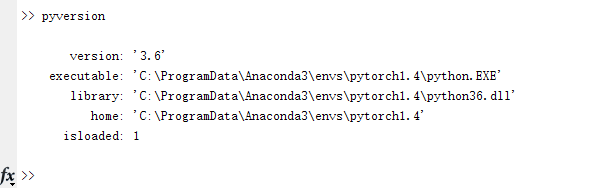由此可见，matlab 是可以识别python版本的。如果你的matlab的命令行里面输入了pyversion，发现并没有找到python,那可能是python的环境变量没有添加进去，添加环境变量步骤如下：

右击我的电脑-----------属性--------高级系统设置------------环境变量———系统变量————path------新建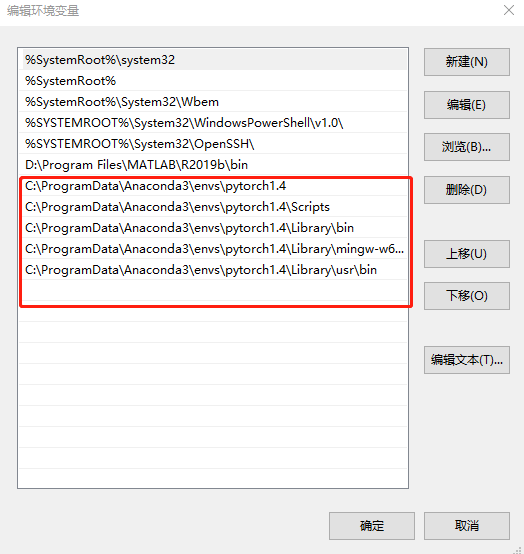根据自己的python路径，添加环境变量即可。

2、matlab和python的交互测试

（1）matlab调用python：

在python中写入函数：

# myfun.py
def test():
print('Hello, Matlab!')

c = a + b
return c

将Matlab切换到myfun.py所在的目录，调用Python函数（非常重要，本人踩过雷，）

在matlab的命令行输入：py.myfun.test()

亲测如下：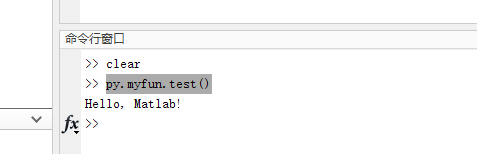至此，matlab调用python成功。

（2）python调用matlab

首先在matlab中配置好python的环境
1、在matlab软件安装路径下，找到文件目录“./extern/engines/python”，具体如下所示（红色框选的文件为原始存在的文件）：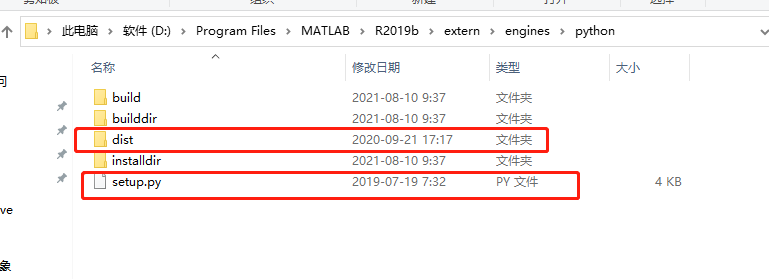2、以管理员身份运行cmd(必须是管理员身份运行，踩过雷，不然会拒绝访问)

切换到python文件夹下：

cd /d D:\Program Files\MATLAB\R2019b\extern\engines\python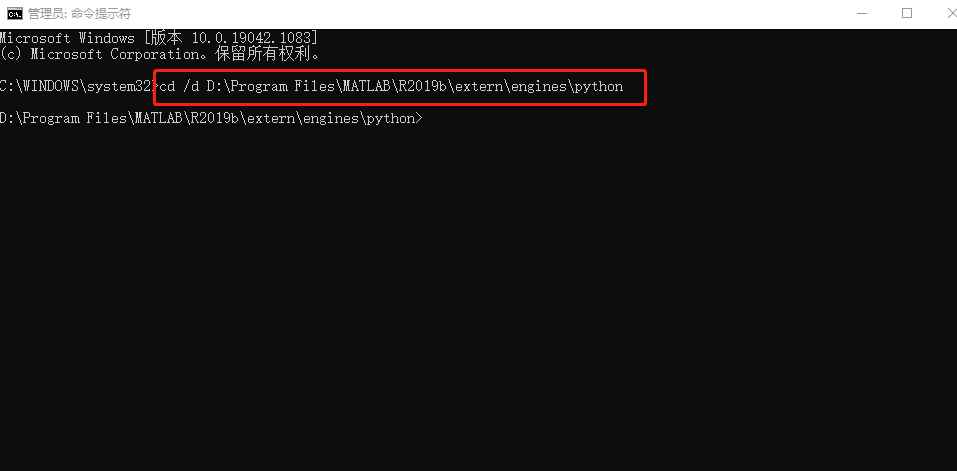然后依次执行下面的代码：

python setup.py build --build-base="builddir" install

python setup.py install --prefix="installdir"

python setup.py build --build-base="builddir" install --prefix="installdir"

python setup.py install --user

执行完毕后，将会生成步骤1图中三个没有被红色框框出来的三个文件夹

3，测试代码：

# # coding=utf-8
import matlab.engine
from numpy import *

if __name__ == '__main__':
eng = matlab.engine.start_matlab('MATLAB_R2019b')
A = matlab.double([[1, 2], [5, 6]])
print(type(A), A.size, A)
print(eng.eig(A))
eng.quit()
pass

结果如下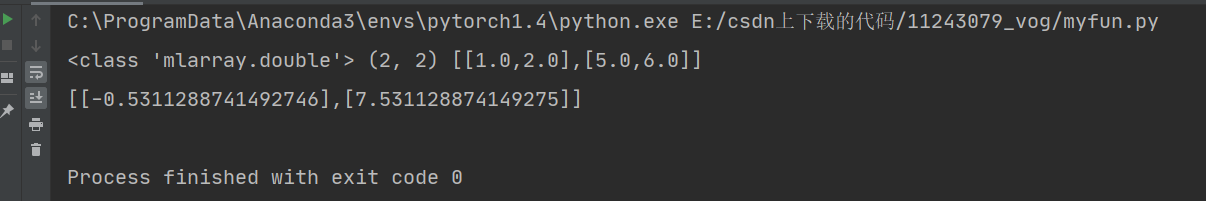至此，大功告成

展开全文xzm961226xzm 2021-08-10 10:48:11
• 一、从matlab调用python 1、先给出官方链接 进入链接后点示例，内容更丰富一些。《Python 库 — 示例》 2、简单说一下环境配置（下面的图片内容来自https://blog.csdn.net/jnulzl/article/details/51170859） 3、...

一、从matlab调用python

强烈建议先看看这B站的视频，正片从中间开始

1、先给出官方链接

进入链接后点示例，内容更丰富一些。《Python 库 — 示例》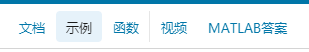2、简单说一下环境配置（下面的图片内容来自https://blog.csdn.net/jnulzl/article/details/51170859）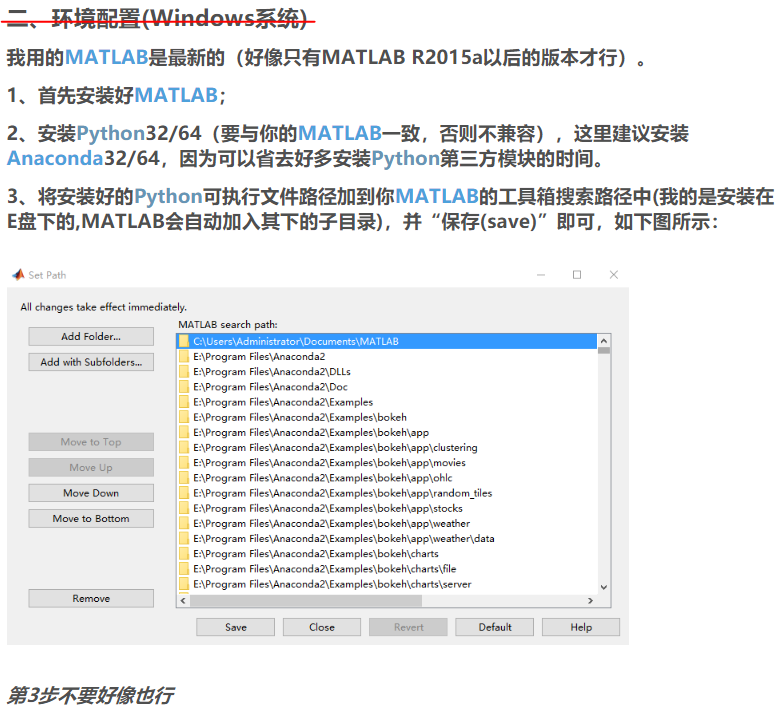3、添加python环境变量以加载模块

如果是将当前文件夹加入到python搜索路径，modpath='';即可。

P = py.sys.path;
if count(P,modpath) == 0
insert(P,int32(0),modpath);
end

修改好路径后，直接使用 import 命令加载 python 模块会产生错误，需采用以下命令：

mod = py.importlib.import_module('untitled'); % 加载 python 模块 untitled.py，这是你自己写的模块文件

当加载了python模块后，修改了该模块内容，就需要重新导入：

4、使用python

在所有python的语法前加一个py.即可
如：import py.numpy
更多可以参考《MATLAB中调用Python》的标题三

三、matlab提供其他语言的接口

展开全文weixin_43469047 2020-03-12 09:31:12

5.76MB weixin_38626179 2021-05-29 01:46:04
• A friend asked me about creating a small web interface that accepts some inputs, sends them to MATLAB for number crunching and outputs the results. I'm a Python/Django developer by trade, so I can han...A friend asked me about creating a small web interface that accepts some inputs, sends them to MATLAB for number crunching and outputs the results. I'm a Python/Django developer by trade, so I can handle the web interface, but I am clueless when it comes to MATLAB. Specifically:

I'd really like to avoid hosting this on a Windows server. Any issues getting MATLAB running in Linux with scripts created on Windows?

Should I be looking into shelling out commands or compiling it to C and using ctypes to interact with it?

If compiling is the way to go, is there anything I should know about getting it compiled and working in Python? (It's been a long time since I've compiled or worked with C)

Any suggestions, tips, or tricks on how to pull this off?

解决方案

There is a python-matlab bridge which is unique in the sense that Matlab runs in the background as a server so you don't have the startup cost each time you call a Matlab function.

from pymatbridge import Matlab

mlab = Matlab(matlab='/Applications/MATLAB_R2011a.app/bin/matlab')

mlab.start()

res = mlab.run('path/to/yourfunc.m', {'arg1': 3, 'arg2': 5})

print res['result']

where the contents of yourfunc.m would be something like this:

%% MATLAB

function lol = yourfunc(args)

arg1 = args.arg1;

arg2 = args.arg2;

lol = arg1 + arg2;

end

展开全文weixin_35411561 2021-07-16 19:23:19
• weixin_33121737 2020-12-29 08:35:14
• 121KB qq_36125564 2020-11-13 12:48:37
• weixin_39695374 2020-11-26 08:05:56
• weixin_39962394 2020-11-21 03:43:55
• weixin_39834406 2020-11-11 14:46:51
• qq_40691682 2021-02-07 12:39:45
• weixin_39622901 2020-11-11 14:09:57
• C语言、Python和MATLAB区别 matlab c语言 python

junchi_yan 2021-10-06 21:57:32
• 【Matlab/Python】Matlab和Python之间的数据传输 数据 matlab python 数据存储

chenghtao 2017-09-13 09:46:38
• weixin_29534463 2021-04-30 03:15:52
• weixin_39932939 2020-11-21 03:43:57
• weixin_40008884 2021-01-14 10:13:52
• weixin_39777018 2020-11-28 23:53:50
• weixin_42504858 2021-01-13 11:30:48
• weixin_39612720 2020-11-23 11:31:43
• weixin_39797264 2020-12-11 13:40:11
• weixin_42373103 2021-03-18 09:54:28
• weixin_28994403 2021-01-11 22:04:40
• python调用matlab文件报错信息为乱码的解决方案 pycharm matlab

qq_37720864 2021-06-22 10:41:25...

python和matlab交互python 订阅matlab 订阅